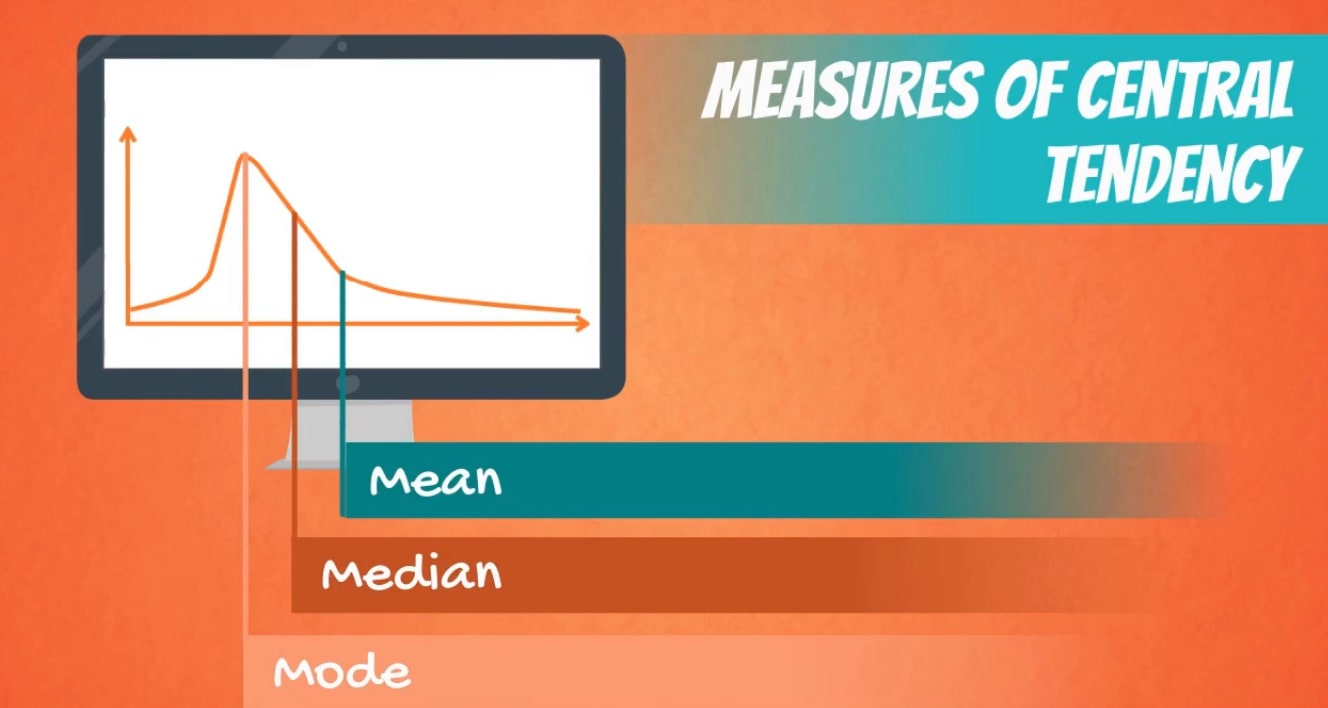# MEASURE OF CENTRAL TENDENCY

Welcome to Class !!

In today’s Mathematics class, We will be looking at the Measures of Central Tendency. We hope you enjoy the class!### CALCULATION OF RANGE, MEAN, MEDIAN AND MODE OF UNGROUPED DATA

##### RANGE

The range of a set of numbers is the difference between the largest and the smallest numbers.

Example: Find the range of the following set of scores: 79, 60, 52, 34, 58, 60.

Solution

Arrange the set in rank order: 79, 60, 60, 58, 52, 34

The range is 79 – 34 = 45

##### THE MEAN

There are many kinds of average. The mean or arithmetic mean, is the most common kind. If there are n numbers in a set, then

Examples

1) Calculate the mean of the following set of numbers.

176   174   178   181   174

175   179   180   177   182

Solution

2) Five children have an average age of 7 years 11 months. If the youngest child is not included, the average increases to 8 years 4 months. Find the age of the youngest child.

Solution

Total age of all five children

= 5 x 7 yr 11 mo

= 35 yr 55 mo

= 35 yr + 4 yr 7 mo

= 39 yr 7 mo

Total age of the four older children

= 4 x 8 yr 4 mo

= 32 yr 16 mo

= 32yr + 1 yr 4 mo

= 33 yr 4 mo

Age of youngest child

= 39yr 7 mo – 33 yr 4 mo

= 6 yr 3 mo

EVALUATION

1) Find x if the mean of the numbers 13, 2x, 0, 5x and 11 is 9. Also, find the range of the set of numbers.

2) A mother has seven children. The mean age of the children is 13 years 2 months. If the mother’s age is included, the mean age rises to 17 years 7 months. Calculate the age of the mother.

#### MEDIAN AND MODEMEDIAN: If a set of numbers is arranged in order of size, the middle term is called the median. If there is an even number of terms, the median is the arithmetic mean of the two middle terms.

Examples

Find the median of a) 15, 11, 8, 21, 17, 3, 8         b) 3.8, 2.1, 4.4, 8.3, 9.2, 5.0.

Solution

a) Arrange the numbers in rank order (i.e. from highest to lowest).

21, 17, 15, 11, 8, 8, 3

There are seven numbers. The median is the 4th number, 11.

b) Arrange the numbers from the lowest to highest.

2.1, 3.8, 4.4, 5.0, 8.3, 9.2

There are six numbers. The median is the mean of the 3rd and 4th terms.

Median = (4.4 + 5.0) /2

= 4.7

MODE: The mode of a set of numbers is the number which appears most often, i.e. the number with the greatest frequency.

Example: Twenty-one students did an experiment to find the melting point of naphthalene. The table below shows the results. What was

a) the modal temperature

b) the median temperature?

temperature (oC)      78    79    80   81   82   83   90

frequency                   1      2       7     5      3     2      1

a) Seven students recorded a temperature of 80o This was the most frequent result.

Mode = 80oC

b) There were 21 students. The median is the 11th If the temperatures were written down in order, there would be one of 78oC, two of 79oC, seven of 80oC, and so on. Since 1 +2 + 7 = 10, the 11th temperature is one of the five 81oCs.

Median = 81o C.

Evaluation

For the following set of numbers:

13, 14, 14, 15, 18, 18, 19, 19, 19, 21

a) state the median, b) state the mode c) calculate the mean.

WEEKEND ASSIGNMENT

1) The number of goals scored by a team in nine handball matches are as follows:  3, 5, 7, 7, 8, 8, 8, 11, 15

Which of the following statements are true of these scores?

• a) The mean is greater than the mode.
• b) The mode and the median are equal.
• c) The mean, median, and mode are all equal.

Use the table below to question 2-5

The table below shows the number of pupils (f) scoring a given mark (x) in attest.

X     2      3      4      5       6     7      8       9       10     11      12

f     3       8      7      10    13  16    15     15      6        2       5

2) Find the mode.

a) 7 b) 8 c) 9     d) 10

3) Find the median.

a) 6 b) 7 c) 8     d) 9

4) Calculate the mean.

a) 6.7    b) 6.8    c) 6.9    d) 6.95

5) Find the range.

1. a) 10 b) 11 c) 9      d) 12

THEORY

1)x, x, x, y represent four numbers. The mean of the numbers is 9, their median is 11. Find y

2) Students at a teacher training college are grouped by age as given in the table below.

Age (years)       20       21       22      23      24      25

Frequency          4         5        10      16      12      3

• a) Find the modal age.
• b) Find the median age.
• c) Calculate the mean age of the students.

We have come to the end of this class. We do hope you enjoyed the class?

Should you have any further question, feel free to ask in the comment section below and trust us to respond as soon as possible.

We have come to the end of this term. It’s been a remarkable journey and we are glad that you have made it this far. For making it this far, we commend you for being resilient, you have taken charge of your education and future.

The Journey still continues though, we are moving on to Second Term. we hope to meet you there.

Are you a Parent? Share your quick opinion and win free 2-month Premium Subscription

Don`t copy text!